## Tuesday, March 29, 2011

### Trying to make an images mosaic with matplotlib

In ImageJ, it is easy to make an images gallery with make montage.Complete particles gallery made with make montage in imagej
The script to produce the particles from the metaphase is here. The end of the code tries to produce a particles (single/overlapping chromomes, nuclei) gallery using pylab.subplot, it is commented because it doesn't work.
I get the answer at pythonvision:

```
import readmagick
import pylab
import os

user=os.path.expanduser("~")
workdir=os.path.join(user,"Applications","ImagesTest","jp","Jpp48","13","DAPI","particules")
file="part1.png"
if __name__ == "__main__":
file="part1.png"
complete_path=os.path.join(workdir,file)
i1=readmagick.readimg(complete_path)
file="part2.png"
complete_path=os.path.join(workdir,file)
i2=readmagick.readimg(complete_path)
file="part3.png"
complete_path=os.path.join(workdir,file)
i3=readmagick.readimg(complete_path)
file="part4.png"
complete_path=os.path.join(workdir,file)
i4=readmagick.readimg(complete_path)
pylab.subplot(2,2,1)
pylab.imshow(i1)
pylab.subplot(2,2,2)
pylab.imshow(i2)
pylab.subplot(2,2,3)
pylab.imshow(i3)
pylab.subplot(2,2,4)
pylab.imshow(i4)
pylab.show() ```
Removing the axis from the displayed images:
Axis can be removed  from the images as follow:
```
pylab.subplot(2,2,1,frameon=False, xticks=[], yticks=[])
```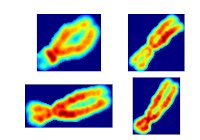click to enlarge

## Friday, March 18, 2011

### Fluorochrome signal calibration in QFISH analysis

It is desirable that independently from the imaging system or from the fluorochrome used (FITC, Cyanine, ...), the intensity of the fluorescent probe depends only on the number of targets in the biological sample.
Spectral calibration:
In order to compare the FISH signal obtained with different fluorochromes as FITC, Cy3, Cy5, the following assay can be performed with conventional material:
The following result was obtained with a leica DMR microscope equiped with a KAF1600 CCD camera:
The data were linearly fitted and the sensitivity of a given fluorochrome was set as the slope of the linear response (gray level per time unit for a give probe concentration). Cy3 yields 713 gray level per 1/10 sec, Cy5:403 gl/time and FITC:297. Thus taking Cy3 as reference: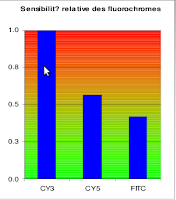Relative sensitivity of fluorescent probe.
Color compensation
In fluorescent microscopy, several fluorochromes are present on a biological sample. The light emitted by each fluorochrome is observed in a spectral window "opened" by a filter in the microscope.

DAPI stains the DNA, with the DAPI block filters (the "blue window""or the blue channel), when you watch in the microscope oculars to see a DAPI stained metaphase, it looks blue. Thus,if you try to watch DAPI stained chromosomes in a green channel (with a FITC band pass filter), you should see nothing: this is true with naked eyes but wrong with a CCD camera with long exposure time. In the following example, images of a DAPI stained metaphase were taken in different spectral channels with different exposure time. Excepted for DAPI, the filters set were from omega filters(alpha vivid serie):
DAPI:blue channel filter A
FITC:green channel alpha vivid XF100-2
Cy3:orange channel alpha vivid XF108-2
Cy5: near infrared channel alpha vivid XF110-2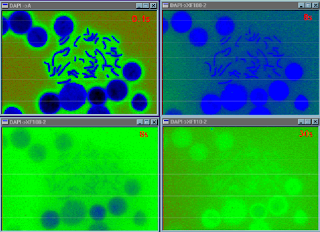DAPI spectral overlap displayed with false color.Top left:DAPI viewed through filter A (0.1s). Top right:DAPI viewed through green channel (8s). Bottom left: DAPI viewed through orange channel (8s).Bottom right:DAPI viewed through deep red channel for 30s.
As the exposure time increases, DAPI is visible in the green channel, in the orange channel and even the near infra red channel after 30 sec of exposure.
The same is true for other fluorochromes, as FITC. The following example is the result of  a FITC labelled telomeric PNA probe hybridizing V79 cell line chromosomes.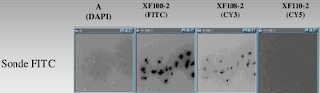FITC spectral overlap in blue, orange and red channels
To address this issue, Castleman (Digital Image processing, Prentice Hall, p556-561), define a color compensation technique relying on linear algebra:

y=ECx+b

where E a diagonal matrix specifying the relative exposure time of each channel and C a matrix accounting for the spectral spread through the channels with cij the proportion of signal  from fluorophore j visible in the channel i.
x  is a 1 by m (m fluorochromes) vector representing an ideal image with no spectral spread.
y is a 1 by m (m fluorochromes) vector representing the observed image with no spectral spread.
b  is a 1 by m vector accounting for the background.
To compute an ideal image with no spectral spread, the following equation has to be solved:
x=C'E'(y-b)
with C' and E' the inverse matrix of C and E.
For example, with four fluorochromes (DAPI, FITC, Cy3, Cy5), the EC matrix was: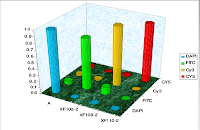graphical representation of the EC matrix

## Monday, March 14, 2011

### Extracting chromosomes from a complex metaphase

Using the previous code on a real metaphase image, chromosomes can be isolated: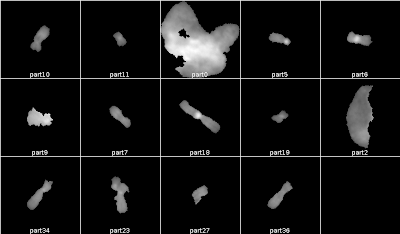Partial gallery of extracted particles (made with make montage in ImageJ)
The gray level image:
The labelled image:
The code of the python script to extract particles from an image is modified quick and dirty such:
```
path='/home/claire/Applications/ImagesTest/JPP60-3/DAPI/'
lab=readmagick.readimg(path+'dapilabel.png')
img=readmagick.readimg(path+'dapi.png')
if __name__ == "__main__":
Particles=extractParticles(img,lab)
for i in range(0,len(Particles)):
file='part'+str(i)+'.png'
readmagick.writeimg(Particles[i].astype(np.uint16), path+file)
```

### Extracting particles from an image: a toy model with numpy

Image segmentation can be performed by an image labelling process. Once segmented, it could necessary to isolate in independant images the labelled regions. Think of chromosomes in a metaphase image, if resolving chromosomes overlapps or karyotyping must be performed.

The following script takes "two images", (in fact two small numpy arrays : img is supposed to be a grey level image and lab, the label image which could be obtained after segmentation) and returns two particles, that is two images corresponding to the labelled regions (1 or 2. 0 corresponds to the background):

The left image corresponds to a labelled image. The two upper images were obtained with extractParticles() and the two lower images were extracted with:
scipy.ndimage.find_objects()

find_objects() doesn't extract the particles properly.

Update 2014-29-01
The code is on gist
If ipython is not installed on your machine, play with it on cloudsagemath.

` `
``` #pseudo 16 bits gray level image
img=np.array([\
[75,167,181,105,33,5,2,2],\
[72,163,193,136,54,10,1,2],\
[54,123,154,118,51,11,2,2],\
[23,54,69,58,33,18,11,4],\
[5,15,27,43,62,69,53,23],\
[5,24,61,104,147,162,124,54],\
[10,54,128,186,220,222,168,72],\
[14,72,169,230,247,238,177,76]],np.int16)
```
```
#pseudo 16 bits label image
lab=np.array([\
[0,1,1,1,0,0,0,0],\
[0,1,1,1,1,0,0,0],\
[0,1,1,1,1,0,0,0],\
[0,1,1,1,0,0,0,0],\
[0,0,0,0,0,2,2,2],\
[0,0,0,2,2,2,2,2],\
[0,0,2,2,2,2,2,2],\
[0,2,2,2,2,2,2,2]],np.int16)
```
```#
#March14, 2011, A script to extract regions (particles) from an array (image)
#Jean-Patrick Pommier
#
import numpy as np
import os
def extractParticles(grayIm,labIm):
''' give a grayscaled and a labelled image, extract the segmented particles
,returns a list of flattened particles'''
#grayIm and labIm should have the same size
def unflattenParticles(flatParticleList):
'''take a list of flat particles and unflat them to yield an image'''
unflatList=[]
lenFlatList=len(flatParticleList)
for i in range(0,lenFlatList):
#get the i particle:current Particle
curPart=flatParticleList[i]#current particle
#x values(col) are stored in the third col (3-1)
colmax=curPart[:,2].max()
colmin=curPart[:,2].min()
#y values(li) are stored in the fourth col (4-1)
limax=curPart[:,3].max()
limin=curPart[:,3].min()
unflatIm=np.zeros((limax-limin+1,colmax-colmin+1),np.int16)
#number of pixels in the particle
nbPixel=len(curPart[:,1])#count how many lines at col=1
for line in range(0,nbPixel):
col=curPart[line,2]
li=curPart[line,3]
pixVal=curPart[line,1]
unflatIm[li-limin,col-colmin]=pixVal
unflatList.append(unflatIm)
return unflatList

sx=grayIm.shape
sy=grayIm.shape
#flatten grayIm
fg=grayIm.flatten()
fl=labIm.flatten()
labmax=fl.max()
#print fg
#print fl
#build two 2D array containing x and y
#of each pixel of the grayIm
ax=np.zeros((sx,sy),np.int16)
ay=np.zeros((sx,sy),np.int16)
#vectorization with numpy may be
#more efficient than two loops
for j in range(0,sy):
for i in range(0,sx):
ax[i,j]=j#filling ax with x=col
ay[i,j]=i#filling ay with y values y=li
#flat arrays of coordinates
fax=ax.flatten()
fay=ay.flatten()
#1D merge graylevel, label and coordinates
#in one 1D array of 4-uplet
extract=np.vstack((fl,fg,fax,fay))
#transpose to watch it easily
eT=extract.T
#create a list of flatten particles
#labIndex takes the value from 1 (the first particle to labmax the\
#label of the last particle
flatParticleList=[]#from Matthieu Brucher
for labIndex in range(1,labmax+1):
flatParticleList.append(eT[eT[:,0]==labIndex])#from Matthieu Brucher
return unflattenParticles(flatParticleList)

if __name__ == "__main__":
Particles=extractParticles(img,lab)
for i in range(0,len(Particles)):
print Particles[i]
print
```
Since that script is supposed to work on true images with a least 1536x1024 pixels, some part of that python script may be optimized with numpy, as:
```
#vectorization with numpy may be
#more efficient than two loops
for j in range(0,sy):
for i in range(0,sx):
ax[i,j]=j#filling ax with x=col
ay[i,j]=i#filling ay with y values y=li
```